Lesson Plan

## Transforming Parabolas Using Vertex Form

• Grade Levels
• Related Academic Standards
• Assessment Anchors
• Eligible Content
• Big Ideas
• Families of functions exhibit properties and behaviors that can be recognized across representations. Functions can be transformed, combined, and composed to create new functions in mathematical and real world situations.
• Mathematical functions are relationships that assign each member of one set (domain) to a unique member of another set (range), and the relationship is recognizable across representations.
• Numbers, measures, expressions, equations, and inequalities can represent mathematical situations and structures in many equivalent forms.
• Patterns exhibit relationships that can be extended, described, and generalized.
• Relations and functions are mathematical relationships that can be represented and analyzed using words, tables, graphs, and equations.
• There are some mathematical relationships that are always true and these relationships are used as the rules of arithmetic and algebra and are useful for writing equivalent forms of expressions and solving equations and inequalities.
• Concepts
• Algebraic properties, processes and representations
• Exponential functions and equations
• Polynomial functions and equations
• Quadratic functions and equations
• Competencies
• Extend algebraic properties and processes to quadratic, exponential, and polynomial expressions and equations and to matrices, and apply them to solve real world problems.
• Represent a polynomial function in multiple ways, including tab les , graphs, equations, and contextual situations, and make connections among representations; relate the solution of the associated polynomial equation to each representation.
• Represent a quadratic function in multiple ways, including tab les , graphs, equations, and contextual situations, and make connections among representations; relate the solution of the associated quadratic equation to each representation.
• Represent exponential functions in multiple ways, including tab les , graphs, equations, and contextual situations, and make connections among representations; relate the growth/decay rate of the associated exponential equation to each representation.

### Objectives

This lesson connects previous experience and knowledge of quadratic functions to a new way of writing quadratic equations. Students will:

• understand what the parameters a, h, and k have to do with the graph of a parabola.

• write the vertex form of a parabola.

• sketch graphs of parabolas given the equation.

• write equations based on real-world problems using the vertex form.

• write their own application problems.

#### Essential Questions

• How can we determine if a real-world situation should be represented by a quadratic, polynomial, or exponential function?

• How do quadratic equations and their graphs and/or tables help us interpret events that occur in the world around us?

### Vocabulary

• Parabola: In a Cartesian coordinate system, the graph that represents the general equation y = ax2 + bx + c and is a conic section that is the intersection of a right circular cone and a plane parallel to a generating straight line of that cone.

• Quadratic Function: A function of the form f(x) = ax2 + bx + c, where a, b, and c are real numbers.

• Standard Form: The form of a quadratic function expressed as an equation where a is the coefficient of x2, b the coefficient of x, and c is the constant, commonly y = ax2 + bx + c.

• Vertex Form: The form of a quadratic equation where for functions of the form f(x) = ax2 + bx + c, parameters a, h, and k in y = a(x h)2 + k. determine characteristics of the parabola that represents the function.

• Stretch Factor: The multiplicative factor by which a graph is compressed or stretched; i.e., a factor of 2 compress the graph, increase each y-coordinate by a factor of 2 relative to the original graph.

• Horizontal Shift: The number of units a graph is moved to the right or left.

• Vertical Shift: The number of units a graph is moved up or down.

• Maximum: A point, (a, f(a)) where f(a) is greater than all other y-values in the range.

• Minimum: A point, (a, f(a)) where f(a) is less than all other y-values in the range.

• Compressed: A graph that has a stretch factor greater than 1; compared to its parent function, the graph looks as if it has been squeezed together, horizontally.

• Vertex of a Parabola: The point on a parabola (either a maximum or minimum, depending on the sign of a) at which the parabola either stops increasing or stops decreasing.

• Transformation: Changing the shape of a parabola by multiplying or dividing a, reflections, or shifting it horizontally and/or vertically. [IS.1 - Language Function]

### Duration

120–180 minutes [IS.2 - All Students]

### Prerequisite Skills

Prerequisite Skills haven't been entered into the lesson plan.

### Related Materials & Resources

The possible inclusion of commercial websites below is not an implied endorsement of their products, which are not free, and are not required for this lesson plan.

### Formative Assessment

• View
• Evaluation of group activities is most effective when the teacher participates in the discussions and poses questions and challenges to student observations. In class discussions, ask leading questions when a student makes an incorrect statement. [IS.23 - All Students]

• Exit Ticket activity (M-A2-2-1_Lesson 1 Exit Ticket.doc[IS.24 - All Students] uses a real-world example of an approximation of a parabola. Students’ solutions will reflect the understanding of how to apply the components of the graph to find the corresponding equation.

### Suggested Instructional Supports

• View
Active Engagement, Modeling, Explicit Instruction

[IS.22 - All Students]

 W: In this lesson, students will advance their ability to represent a quadratic function in terms of the shape and parameters of its graph and to use the vertex form in writing quadratic equations and solving problems. H: The quadratic function y = x2 + 6x + 8 presents a challenge for students to identify its vertex through simple inspection. Recognizing that the positive a term makes its graph open above gives them a starting point. Having students share their thoughts on the nature and location of the vertex provokes thinking about the behavior of the function. E: The human parabola activity helps students, both individually and collectively, think about the locations of coordinates of quadratic functions in ways that are visual as well as tactile. Students must calculate corresponding y-coordinates from given values of x, and then find each position in relation to the vertex. R: Students use the Steps to Equation Writing Worksheet to examine the dimensions of the half-pipe and locate the coordinates of the vertex, x- and y-coordinates. This process helps them translate the physical structure into a representation of a quadratic function. E: Lesson 1 Exit Ticket evaluates students’ understanding of using the dimensions of a physical object (suspension bridge) to determine the components of the quadratic function it represents. T: Multiple learning styles are addressed through making a human parabola. For students who have difficulty plotting x and y coordinates, use only integral ordered pairs. To assist students who are challenged by functions with binomials raised to the second power, simplify expressions before assigning. O: This lesson is necessary for students to become familiar with the vertex form of a parabola. They cannot use the completing-the-square technique without knowing why they need to convert from standard form to vertex form. This lesson illustrates how easy it can be to write the equation of a parabola, state what the transformations are, or see the vertex in the equation. In the following lessons, more will be covered that will make this technique (working with the vertex form) useful.

 IS.1 - Preparation Consider using graphic organizers (e.g., Frayer Model, Verbal Visual Word Association, Concept Circles) to review key vocabulary prior to the lesson. IS.2 - All Students Consider preteaching the concepts critical to this lesson, including the use of concrete representations for the concepts and skills. Throughout the lesson (based upon the results of formative assessment), consider the pacing to be flexible to the needs of the students. Also consider the need for reteaching and/or review both during and after the lesson as necessary. IS.3 - Struggling Learners Consider supplying struggling students a copy of an algebraic equation in standard form for the quadratic function and other forms of quadratic function representation (e.g. the graph and numeric table of the function). Using multiple representations to make connections helps deepen understanding and connect with prior learning. IS.4 - All Students Consider continuing to utilize multiple representations of the function (numeric table, graph and standard form of the algebraic equation) which help strengthen a student’s understanding of the quadratic relationship. IS.5 - All Students Consider pre-teaching transitions by using a graphing calculator as a tool to investigate translations without needing the cognitive demand of graphing procedures. Also provide a copy of the function and related translations  with space available for notes (or include  notes  depending on the needs of the student). IS.6 - Struggling Learners Consider allowing the use of calculators so that a struggling student can focus on the characteristics of the parabola, not the calculations involved in finding the ordered pairs that satisfy the function. IS.7 - All Students Consider providing students with a copy of the function’s graph, numeric table and algebraic equation in standard form for their group. This will provide a visual and symbolic representation of the concrete representation that is being created. IS.8 - All Students Consider using a graphic organizer to compare/contrast the functions. IS.9 - All Students Consider providing students with a copy of the function’s numeric table and verbal description of the relationship created by the function. Continue to reference the table and verbal descriptions as the students create the function’s graphs on the calculator so that connections and expected student understanding are made explicit. IS.10 - All Students Consider creating questions before teaching the lesson that will assess and advance students depending on their level of need without lowering the cognitive demand of the activity. IS.11 - All Students Consider using manipulatives that mirror the shape of the function’s graph so that students can physically manipulate the translation or reflection. Remember to make connections to between concrete, representational and abstract forms of relationship explicit for the students. IS.12 - Struggling Learners Consider providing struggling students a copy of the completed graphic organizer. IS.13 - Struggling Learners Consider providing the struggling learner a copy of the graph for each of the function. Make the connection between the graph’s characteristics and the algebraic equation’s characteristics (related to the translations and vertex) explicit. IS.14 - Struggling Learners Consider providing a picture or other visual of the ramp that highlight’s the parabolic features (overlay a coordinate system on top of the picture or visual) to aid the struggling student as he/she strives to make connections between a real life object and the graphical representation of the parabola. IS.15 - All Students Consider listing the questions that could be used to support students as they write the equation for the parabola. IS.16 - All Students Consider reviewing the steps involved in solving this type of equation before teaching the lesson so the student’s focus can be on the dilation, translation and possible reflection instead of the steps for solving the equation. IS.17 - All Students Consider grouping students ahead of time so that the groupings can be done intentionally by the instructor. IS.18 - All Students Consider providing students with a picture or diagram representation of the problem situation and scaffolding parts of the algebraic equations so that students are translating smaller bits of the problem into algebraic equation that represents the problem. As students gain proficiency in their ability to create the algebraic equations, remove some of the given components. Continue to work on removing scaffold based on student needs until the student can model the problem without the supports. IS.19 - All Students Consider using multiple representations (graphs, numeric tables, language descriptions and algebraic equations) to support students in their attempts to understand the dilation factor. IS.20 - All Students Consider providing a graph of the function along with the vertex and the point. Place the parent quadratic function’s graph on the same coordinate system so that students can see the translations, dilations and reflections that have taken place. IS.21 - Struggling Learners Consider providing notes to the struggling learner so that the focus can be on learning about quadratic functions, not note taking. IS.22 - All Students Also consider the use of Think-Alouds on the part of the students and the teacher. IS.23 - All Students Consider planning this lesson while thinking about the common preconceptions and misconceptions that students will bring. This allows the instructor to plan strategic points for formative assessment strategies in the lesson and to plan answers to the questions that are likely to be asked. For additional information about questions that the instructor can ask the student (assessing and advancing questions), please read the Teachers’ Desk Reference: Essential Practices for Effective Mathematics Instruction by going to http://www.pattan.net/category/Resources/PaTTAN%20Publications/Browse/Single/?id=4e1f51d3150ba09c384e0000 IS.24 - All Students For additional information on formative assessment, please visit www.pdesas.org and click on the “Fair Assessments” tab

### Instructional Procedures

• View

After this lesson, students will be able to write the vertex form of a quadratic function: y = a(x h) + k. They will know what the parameters a, h, and k have to do with the graph of a parabola. They will be able to look at the equation and instantly see the vertex, which gives them the minimum or maximum value of the graph. Students will be able to sketch graphs of parabolas quickly using the vertex form and will be able to write the equation given the graph. This lesson familiarizes students with the vertex form of a parabola to prepare them to be successful in the next two lessons. In addition, students need to see a purpose for what they are learning, and since quadratic relationships and parabolas happen all around us, they will be more motivated to learn the content. After this lesson, students will understand that the vertex form of a parabola can be more useful than the standard form, and this will set them up to be successful in the following lessons.

Students should begin this lesson by reviewing concepts of minimum, maximum, vertex, and axis of symmetry as they relate to parabolas. Hand out copies of the Entrance Ticket for students to complete (M-A2-2-1_Entrance Ticket.doc).

Think-Pair-Share

Think about the quadratic function y = x2 + 6x + 8. [IS.3 - Struggling Learners] Without graphing it, how could you determine the coordinates of the vertex?”

For the first minute, tell students to keep their pencils down. For the second minute, have them write their ideas of how to find the vertex. When time is up, pair students to share their thoughts with each other. Bring the class together and write all the ideas on the board.

If students struggle coming up with ideas, put the graph on the board.

We know that we can look at the graph and see where the vertex is. But algebraically, how could we determine the coordinates?”

Pause for student ideas and responses.

What is so special about the vertex? What are the characteristics of the vertex?” [IS.4 - All Students] Students might mention the vertex is either the lowest (minimum) or highest (maximum) point on the graph; the vertex is in the middle.

This lesson is going to introduce us to a new way of writing quadratic functions.”

Activity 1: The Human Parabola [IS.5 - All Students]

Take students to a large area (hallway, cafeteria, gym, outside). Do not allow calculators, [IS.6 - Struggling Learners] as students should be able to do the math in their head or on a piece of paper. Place the ropes on the floor as the x- and y-axes. Place students into groups of 5. They are going to be the coordinates of a parabola.

• The first group is going to be y = x2. One student should be the vertex while the other students represent two points on each side of the vertex (example: x = −2, −1, 1, and 2). [IS.7 - All Students] Students will be “connected” with a string.

• The second group is going to be y = (x – 4)2 and then y = (x + 4)2. They should use the same x-values that the first group did.

• The third group is going to be y = x2 + 1 and then y = x2 – 1.

• The fourth group is going to be y = −x2. Some students may come up with different answers. Some students might say that when x = 2, y is 4 while others would say y is −4. This is a good time to discuss order of operations.

• The fifth group is going to be y = 3x2 and then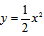.

• Any students not in a group will write everyone’s observations after each equation is graphed. Have them write on poster paper (one for each equation) so you can hang the results in the classroom after the activity.

• Group #1 is always going to stay on the axes. Group #2 will graph their first equation and the entire class should discuss their observations.

• Some questions to ask after each new parabola is graphed are: “What is the difference between the original parabola (y = x2) and the new one? [IS.8 - All Students] (horizontal shift of 4 units right; horizontal shift of 4 units left; vertical shift of 1 unit up; vertical shift of 1 unit down; reflection across the x-axis; vertical stretch by a factor of 3; vertical compression by a factor of 2) What are the coordinates of the vertex of the new parabola? [(0, 4); (0, -4); (0, 1); (0, -1); (0, 0); (0, 0); (0, 0)] Are the shapes of the parabolas the same?” (The shapes are essentially the same, except for the last two which are compressions/stretches, but still retain essentially the same parabolic shape.)

• Group #2 will graph the second equation with discussion to follow. Do this until all equations have been graphed and discussed. Go back to the classroom and hang the posters at the front of the class.

• Can anyone draw any conclusions from the Human Parabola activity? If not, that is okay; we are going to do another activity that may help you understand.”

Activity 2: Individual and Pair Work

Hand out the Parabola Exploration Worksheet (M-A2-2-1_Parabola Exploration Worksheet.doc).

Using your graphing calculator and observations from our first activity, explore what happens to a parabola when the equation changes.” [IS.9 - All Students]

Have students work on this worksheet on their own for a while and then have them work in pairs.

What are some things you noticed that happened to the original graph of y = x2 when the equation was written a little differently?” Discuss the worksheet with the class. [IS.10 - All Students]

Write the vertex form of the quadratic equation on the board: y = a(xh)2 + k. Discuss how the graph of the parabola is affected as the values of a, h, and k are changed.

The vertex of a parabola is at point (h, k). In the equation y = (x − 4)2 + 3, the vertex is at point (4, 3). What happens if we change the 3 to a bigger number?” (The vertex shifts up.) “What happens if we change the (x − 4) to (x + 4)?” [IS.11 - All Students] (The vertex shifts left eight spaces.)

Discuss with students how the parabola is affected with changes in the value of a.

Ask the fifth group (from Activity 1) how its parabola changed between y = 3x2 and. (y = 3x2 was skinnier than, or that as a got smaller the parabola got wider)

How does the parabola change if a is negative?” Wait for responses. (graph gets skinnier, turns upside down, etc.) Ask group 4 (from Activity 1) how the equation y = −x2 looked once it was graphed. (The parabola opened facing down.) “That’s right. When a is positive, the parabola opens upward; when a is negative it opens downward.” Another way of saying this is “When a is positive the vertex is the bottom of the parabola. When a is negative the vertex is the top of the parabola. Is it a minimum or maximum point?”

Distribute the graphic organizer (M-A2-2-1_Graphic Organizer.doc). [IS.12 - Struggling Learners] Help students fill in the blanks for the missing parameters of the vertex form. A key is provided for the graphic organizer (M-A2-2-1_Graphic Organizer KEY.doc). Note that the minimum and maximum occurs at the vertex. The maximum and minimum value is the y-coordinate.

Remind students how to determine/locate the x-value of the vertex. Students need to first find the xh part of the quadratic function in the given equation; since there is a minus sign in front of the h, the x-value of the vertex is always the opposite of what is in parentheses. If the equation has (x – 3)2, then the x-value of the vertex is 3 (NOT negative 3). If the equation has (x + 3)2, then the x-value of the vertex is −3. Also remind students how to determine/locate the y-value of the vertex: look for k.

Activity 3: Mini-Whiteboards

Place the following equations on the board. One equation at a time, have students write the vertex of each parabola on their whiteboard. [IS.13 - Struggling Learners] Give them a simple yes or no. They can go on to the next equation if they get a yes; if they get a no, they need to try again.

1. y = x2 + 3

2. y = (x − 5)2

3. y = −x2

4. y = 3(x + 2)2 − 1

5. y = (x − 3)2 + 4

6. y = (x + 8)2 + 11

7.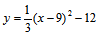8. y = − (x + 4)2 − 3

9. y = (x + 2)2

10. y = −x2 − 4

Answer Key:

1. (0, 3)

2. (5, 0)

3. (0, 0)

4. (−2, −1)

5. (3, 4)

6. (−8, 11)

7. (9, −12)

8. (−4, −3)

9. (−2, 0)

10. (0, −4)

Example:

Gather the class together and ask, [IS.14 - Struggling Learners] “How many of you have tried skateboarding or rollerblading? Have you ever seen a ramp the skaters use to do tricks, called a half-pipe?” Show picture of a half-pipe (M-A2-2-1_Half Pipe.doc). “What does the shape remind you of?” (parabola)

Today you are going to do the math behind designing a half-pipe by writing the equations of the parabolic path half-pipes make. Let’s take a look.” Show the half-pipe as parabola picture (M-A2-2-1_Half Pipe.doc).

How would we begin writing the equation for this parabola? What are some things we learned.” (Students should come up with vertex or stretch factor; if not, give them some hints.) [IS.15 - All Students]

Put the Steps for Writing Equation worksheet (M-A2-2-1_Steps for Writing Equation.doc) on the board or document camera for students to copy down as notes. “Here we have a half-pipe. It is 20 feet long and 8 feet tall. In the middle is a parabola. We are going to write the equation for this parabola.” Point out the parabola and dimensions of the half-pipe on the board.

The first thing we are going to do is turn the picture into a graph by adding x- and y- axes. These can be placed anywhere, but today we’re going to put them at the bottom left of the drawing.” Add or point out axes on the drawing.

Now we are going to label some points on the graph. What points can we label?” Wait for responses. Responses may include the origin, vertex of the parabola, etc.

Let’s go on to the next step. We can label the origin, since we know that it is always at (0, 0). We can see that the vertex of the parabola is one foot up from the x- axis and in the middle of the 20 foot half-pipe. So we can tell that the vertex is at point (10, 1). We can also see one of the points on the parabola is at the top of the half-pipe which is eight feet tall and two feet in from the edge, making it point (2, 8).”

We are going to label the origin, the parabola’s vertex and the point on the parabola.” Label or point out origin (0, 0); vertex (10, 1); and point on parabola (2, 8).

We can use these points to find the equation of the parabola. The first step is to put the vertex (10, 1) into the vertex form of the equation.” Write the vertex form of a parabola on the board y = a(xh)2 + k on the board. Then replace h with 10 and k with 1, resulting in equation y = a(x – 10)2 + 1.

We have completed almost all of the equation, only the stretch factor, a is missing. We will do that by substituting for our other point on the parabola, (2, 8), for x and y. Write the equation, 8 = a(2 – 10)2 +1 on the board. [IS.16 - All Students]

Now we solve for a.

8 = a(2 – 10)2 +1
7 = a(-8)2
7 = a(64)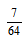= a
We put a back into the vertex form of a parabola and we have the equation for the half-pipe.” Write equation y = (x – 10)2 + 1 on the board.

Activity 4: Group Work [IS.17 - All Students]

Separate the class into groups of three to five. Tell students to keep their notes out for reference. They are going to solve the following two problems. Each person in the group has to place their axes in a different spot. Students will write equations modeling the two problems. When everyone has completed the task, they will pass their equations around to check each other’s work.

Problem #1: Lucy was at the zoo watching the spider monkeys play in the trees. She read that spider monkeys usually stay in trees that reach 150 feet into the air and can swing between trees that are 80 feet apart. [IS.18 - All Students] The bottom of their swing is 60 feet off the ground.

Problem #2: A football was on the ground, and Tony decided to give it a good kick. It landed 100 feet from where he kicked it. While in the air, it reached a height of 125 feet.

Ask the class the following questions for each of the problems above:

What is one similarity among all of your equations?” Students should notice that the stretch factor is the same in all the equations. [IS.19 - All Students]

What is one difference among all of your equations?” Students should notice that the coordinates of the vertex are different in each equation.

What is one way to check and see that your equations represent the same parabola?” (Compare the values of a.)

Activity 5: Individual Work

For each problem, find the equation of the parabola that has the specified vertex and that goes through the given point.” [IS.20 - All Students]

1. Vertex (2, 1) and has point (4, 13)

2. Vertex (−4, −2) and has point (6, −27)

3. Vertex (6, −3) and has point (0, −75)

4. Vertex (−1, 5) and has point (−3, 7)

Answer Key

1. y = 3(x – 2)2 +1

2. y =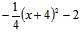3. y = -2(x – 6)2 – 3

4. y =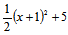Have students write their answers on the board and discuss any issues with confusion or difficulty.

Hand out the Lesson 1 Exit Ticket (M-A2-2-1_Lesson 1 Exit Ticket.doc and M-A2-2-1_Lesson 1 Exit Ticket KEY.doc) to evaluate students’ understanding.

Routine: Group and partner work is used throughout so that students can help each other. Emphasis should be placed on communicating mathematical ideas with specific vocabulary words appropriate to the concepts. The lesson requires accurate note-taking skills to enhance the learning experience while creating a useful resource (notes). [IS.21 - Struggling Learners]

Alternate Lesson Suggestions: This lesson can be covered in two days if so desired. If you wish to cover this lesson over two days, the suggested method is to cover up to and including Activity 3 the first day, then use the Example to reintroduce the topic, then cover Activities 4 and 5 the next day.

Extension:

### Related Instructional Videos

Note: Video playback may not work on all devices.
Instructional videos haven't been assigned to the lesson plan.
DRAFT 08/31/2011Please wait...